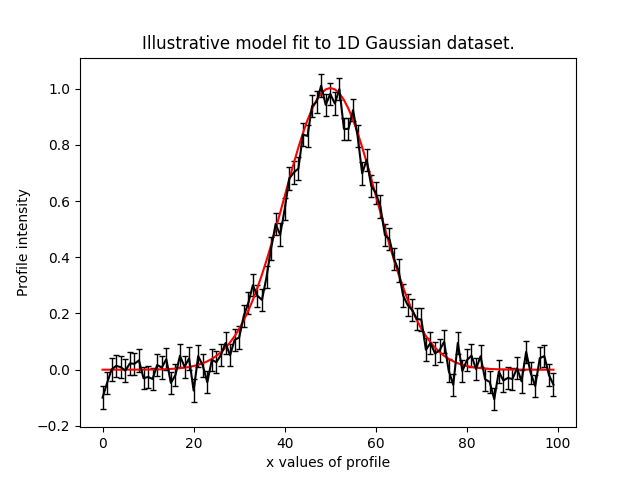# Pyautofit

PyAutoFit: Classy Probabilistic Programming
Alternatives To Pyautofit
Sherpa137
2 days ago471gpl-3.0Python
Fit models to your data in Python with Sherpa.
Polychordlite65
3 months ago18otherFortran
Public version of PolyChord: See polychord.co.uk for PolyChordPro
Machinelearningstatistics54
2 years ago1bsd-3-clauseJupyter Notebook
Machine learning and statistics for physicists
Pyautofit49352 days ago278June 29, 202234mitPython
PyAutoFit: Classy Probabilistic Programming
Mistree33
2 months ago2mitPython
A python package for constructing and analysing minimum spanning trees.
Anki_science22
3 years agoJupyter Notebook
Anki decks for physics, astronomy, computer science, machine learning, and statistics.
Nmmn17
3 months ago1mitPython
Miscellaneous methods for: astronomy, dealing with arrays, statistical distributions, computing goodness-of-fit, numerical simulations and much more
Rcosmo15
8 months ago5December 01, 2021agpl-3.0R
Handle and Analyse Spherical, HEALPix and Cosmic Microwave Background data on a HEALPix grid.
Uw Astr598 W1813
5 years agobsd-2-clauseJupyter Notebook
ASTR 598: Astro-statistics and Machine Learning
Popsynth1319 months ago27June 13, 2022gpl-3.0Python
A generic flux/parameter population synthesis code
Alternatives To Pyautofit
Select To Compare

Alternative Project Comparisons

# PyAutoFit: Classy Probabilistic Programming

PyAutoFit is a Python based probabilistic programming language for the fully Bayesian analysis of extremely large datasets which:

• Makes it simple to compose and fit multi-level models using a range of Bayesian inference libraries, such as emcee and dynesty.
• Handles the 'heavy lifting' that comes with model-fitting, including model composition & customization, outputting results, model-specific visualization and posterior analysis.
• Is built for big-data analysis, whereby results are output as a sqlite database which can be queried after model-fitting is complete.

PyAutoFit supports advanced statistical methods such as graphical and hierarchical models, model-fit chaining, sensitivity mapping and massively parallel model-fits .

## Getting Started

The following links are useful for new starters:

## Why PyAutoFit?

PyAutoFit began as an Astronomy project for fitting large imaging datasets of galaxies after the developers found that existing PPLs (e.g., PyMC3, Pyro, STAN) were not suited to the model fitting problems many Astronomers faced. This includes:

• Efficiently analysing large and homogenous datasets with an identical model fitting procedure, with tools for processing the large libraries of results output.
• Problems where likelihood evaluations are expensive (e.g. run times of days per model-fit), necessitating highly customizable model-fitting pipelines with support for massively parallel computing.
• Fitting many different models to the same dataset with tools that streamline model comparison.

If these challenges sound familiar, then PyAutoFit may be the right software for your model-fitting needs!

## API Overview

To illustrate the PyAutoFit API, we'll use an illustrative toy model of fitting a one-dimensional Gaussian to noisy 1D data. Here's the `data` (black) and the model (red) we'll fit:We define our model, a 1D Gaussian by writing a Python class using the format below:

```class Gaussian:

def __init__(
self,
centre=0.0,        # <- PyAutoFit recognises these
normalization=0.1, # <- constructor arguments are
sigma=0.01,        # <- the Gaussian's parameters.
):
self.centre = centre
self.normalization = normalization
self.sigma = sigma

"""
An instance of the Gaussian class will be available during model fitting.

This method will be used to fit the model to data and compute a likelihood.
"""

def model_data_1d_via_xvalues_from(self, xvalues):

transformed_xvalues = xvalues - self.centre

return (self.normalization / (self.sigma * (2.0 * np.pi) ** 0.5)) * \
np.exp(-0.5 * (transformed_xvalues / self.sigma) ** 2.0)
```

PyAutoFit recognises that this Gaussian may be treated as a model component whose parameters can be fitted for via a non-linear search like emcee.

To fit this Gaussian to the `data` we create an Analysis object, which gives PyAutoFit the `data` and a `log_likelihood_function` describing how to fit the `data` with the model:

```class Analysis(af.Analysis):

def __init__(self, data, noise_map):

self.data = data
self.noise_map = noise_map

def log_likelihood_function(self, instance):

"""
The 'instance' that comes into this method is an instance of the Gaussian class
above, with the parameters set to values chosen by the non-linear search.
"""

print("Gaussian Instance:")
print("Centre = ", instance.centre)
print("normalization = ", instance.normalization)
print("Sigma = ", instance.sigma)

"""
We fit the ``data`` with the Gaussian instance, using its
"model_data_1d_via_xvalues_from" function to create the model data.
"""

xvalues = np.arange(self.data.shape)

model_data = instance.model_data_1d_via_xvalues_from(xvalues=xvalues)
residual_map = self.data - model_data
chi_squared_map = (residual_map / self.noise_map) ** 2.0
log_likelihood = -0.5 * sum(chi_squared_map)

return log_likelihood
```

We can now fit our model to the `data` using a non-linear search:

```model = af.Model(Gaussian)

analysis = Analysis(data=data, noise_map=noise_map)

emcee = af.Emcee(nwalkers=50, nsteps=2000)

result = emcee.fit(model=model, analysis=analysis)
```

The `result` contains information on the model-fit, for example the parameter samples, maximum log likelihood model and marginalized probability density functions.

## Support

Support for installation issues, help with Fit modeling and using PyAutoFit is available by raising an issue on the GitHub issues page.

We also offer support on the PyAutoFit Slack channel, where we also provide the latest updates on PyAutoFit. Slack is invitation-only, so if you'd like to join send an email requesting an invite.

Popular Statistics Projects
Popular Astronomy Projects
Popular Data Processing Categories
Related Searches

Get A Weekly Email With Trending Projects For These Categories
No Spam. Unsubscribe easily at any time.
Python
Bash
Statistics
Astronomy
Mcmc
Bayesian Inference
Statistical Analysis
Probabilistic Programming
Bayesian Methods